American Journal of Nano Research and Applications
Volume 3, Issue 3, May 2015, Pages: 66-70

The Cantorian Monadic Plasma behind the Zero Point Vacuum Spacetime Energy

Mohamed S. El Naschie

Dept. of Physics, University of Alexandria, Alexandria, Egypt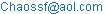Mohamed S. El Naschie. The Cantorian Monadic Plasma behind the Zero Point Vacuum Spacetime Energy. American Journal of Nano Research and Applications. Vol. 3, No. 3, 2015, pp. 66-70. doi: 10.11648/j.nano.20150303.16

Abstract: Stimulated by the recent work on quarks-gluons plasma we present E-infinity theory in the form of Cantorian monadic plasma and proceed from there to a general explanation of the Casimir effect and dark energy as a zero point vacuum energy which could be utilized via advanced nanotechnology to build a clean energy reactor with near to unlimited capacity.

Keywords: Quark-Gluon Plasma, E-Infinity, Cantorian Spacetime, Monadic Cantorian Plasma, Highly Structured Rings, Casimir Effect Dark Energy, Zero Point Vacuum Energy

1. Introduction

In a lucidly written and beautifully illustrated Scientific American feature article by R. Ent, T. Ullrich and Raju Venugopalan they wrote :

"When the cosmos was young, it was too hot for atoms or even stable protons and neutrons to form. Quarks and gluons buzzed around freely in a roiling swarm. Accelerators on Earth recently succeeded in replicating this state, called a quark-gluon plasma by smashing atomic nuclei together at near light speed. By studying the plasma as it cools, physicists can learn not just about the behaviour of quarks and gluons but also about the early evolution of our universe"

Here the present author may add that this is as near as mainstream physics [1-3] can come to a similar view point to that of our Cantorian spacetime [4-9] and our approach leading to the ordinary and dark energy density of the cosmos and that in a superb agreement with measurement and cosmic observations .

The present work aims at putting the highly intuitive picture of quark –gluon plasma  into a relation to Cantorian spacetime which obviously has the advantage of a stringent mathematical formulation of inert transfinite set theory . Thus in a sense we are translating the rather mainstream but highly intuitive picture of the said plasma  to the language of self similar random Cantor sets and its associated highly structured golden mean rings of E-infinity theory [4-9]. The road we are taking here stretches from quantum set to the theories of extra spacetime dimensions and noncommutative geometry [10-15].

*) This paper is dedicated, in deep belated respect, to the memory of the imaginative inventor N. Tesla who died in 1943.

In anticipation of the next section we should mention that in the Cantorian E-infinity space picture, elementary particles and spacetime are made of each other via the zero set representing the first image of the quantum particle monad and the quantum wave monad which is the cobordism, i.e. the surface of the quantum particle monad . The common cobordism of particle and wave then arises naturally as a random multi fractal with the same expectation topology equal to the cobordism of the quantum wave [4-9]. This multi-fractal is thus nothing else but the quantum Cantorian monad which gives rise to Cantorian quantum spacetime itself [4-6] (see also Fig. 1). This situation may seem superficially complex but it is not once it is elaborated upon via von Neumann-Connes dimensional function of noncommutative geometry [4-9], which we do next.

This is basically a two dimensional projection in which each of the larger balls (circles) are a zero set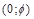representing the quantum particle while the surface (circumference) represents the empty set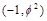which in turn represents the quantum wave. This wave is then surrounded by an infinite hierarchy of smaller (fractal) spheres (surfaces) which may be seen as the emptier set, i.e. the surface of the empty set quantum wave. Remarkably the average set of all zero and empty sets is an expectation value equal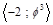. In other wordsis our quantum spacetime which is the cobordism of the quantum wave which in turn is the cobordism of the quantum particle floating and propagating with the help of its wave in our Cantorian E-infinity spacetime. It is likewise remarkable that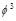is simultaneously equal to the topological Casimir force as well as the topological mass of the ordinary energy of spacetime. Thus all matter and energy manifestations in our cosmos are essentially a manifestation of the ero point energy of the vacuum of spacetime. To obtain Einstein maximal energy density we just need to find first the topological energy density by adding Kaluza-Klein D = 5 toof the spacetime vacuum and find the fractal Kaluza-Klein dimension 5 +then multiply this with the average Cantorian interval speed of light c =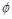squared. The result is (5 +)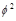= 2. Inserting in Newton’s kinetic energy one finds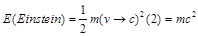exactly as should be. The preceding explanation amounts to a paradigm shift in physics where the totally empty vacuum of spacetime is taken as fundamental and everything else is derivable from it. To prove this point was a dream of Serbian American inventor N. Tesla who died in 1943 as well as Soviet physicist A. Zakharof. In fact in his later years Nobel Laureate J. Schwinger was a champion of cold fusion which comes very near to our present concept of a Casimir-nano energy reactor.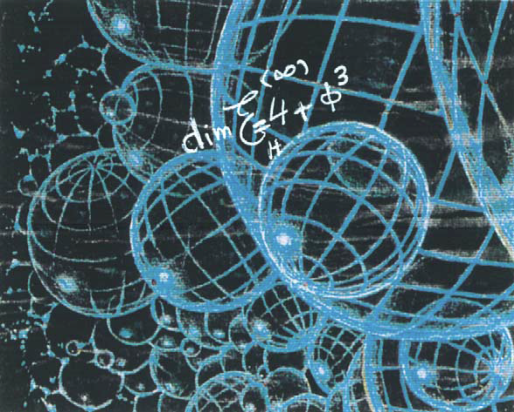Fig. 1. Cantorian spacetime of E-infinity theory which is considered here to model our actual spacetime may be envisaged advantageously as in this artist impression.

2. The Dimensional Function

Following A. Connes [7,9,10] one finds that the dimensional function of a Klein-Penrose compactified space [10,12] which is representative of the holographic boundary of our superstring |E8 E8| exceptional Lie groups universe [4,7], [10-13] is given by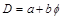(1)

where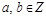and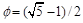. Thus for the zero set we have a = 0 and b =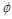and therefore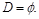(2)

Consequently we have

D(zero set) = (0 ;)                         (3)

Similarly for the empty set we have a = 1 and b =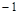which leads to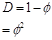(4)

and

D(empty set) =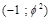.                      (5)

We move next to the monadic interpretation of this result which is basically an extension of the pioneering work of D. Finkelstein on quantum sets  and A. Connes on noncommutative geometry .

3. The Monadic Quantum Mechanics and Quantum Spacetime

Quantum mechanics was initially developed, and contrary to relativity, without any reference to spacetime . However in our E-infinity theory as well as noncommutative geometry and superstrings and M-theory, spacetime is fundamental [4-8], [11-13]. It was reasoned in various previous publications that the monad of the quantum particle is the zero set D(0) = (0,) while the quantum wave monad is basically the surface of D(0), namely by Menger-Urysohn deductive dimensionality theory and von Neumann-Connes theory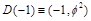[4-]. Now we conclude that the surface of the quantum wave or empty set monad must be an emptier set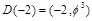. This is remarkable because the average Hausdorff dimension of a quasi multi-fractal made of the zero set and all empty sets, that is to say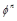for n = 2, 3, 4,….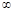is exactly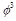because of the well known fact that [4-8]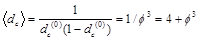(6)

as well as [4-8](7)

and thus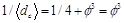(8)

where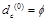is the zero set Hausdorff dimension in the bijection version of von Neumann-Connes dimensional function [4,7,10] (see Fig. 1). The intuitive interpretation of this result will be discussed next.

4. The Spacetime Cantorian Plasma Picture

From the preceding analysis one could develop the following spacetime picture (Fig. 1) and we restrict it to a two dimensional projection of our space with the zero set particle represented by acircle living in an empty set represented bycontainingand finallymay be seen as floating in an infinitely large circle representing an average emptier setwhich is our Cantorian quantum space [4-7]. This is in a sense a Cantorian plasma corresponding in a fuzzy intuitive way to the quark-gluon plasma . Next we look at the application of the previous analysis to dark energy .

5. From Cantorian Monadic Plasma to Ordinary and Dark Energy Density of the Cosmos

Noting that multiplicative Hausdorff "volume" of the zero set in Kaluza-Klein five dimensional space is given by 

Vol(5)(particle) =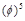,                          (9)

it is easily reasoned that the corresponding energy density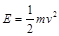(10)

may easily be understood in this case as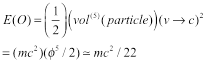(11)

Consequently the dark energy is the difference between the maximal density E(Einstain) = mc2 and E(O) which means 

E(Dark) = mc2(21/22).                   (12)

This could also be obtained directly from the additive volume of the empty set quantum wave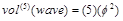(13)

and consequently one finds in a similar way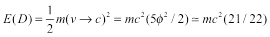(14)

exactly as before [7,8]. Next we discuss the application of our theory to the Casimir effect and more general cases of zero point vacuum energy.

6. The E-Infinity Cantorian Plasma and the Zero Point Vacuum Energy

The Casimir effect is probably the most theoretically and experimentally documented manifestation of the zero point quantum vacuum energy. We take this Casimir effect very seriously which was linked to dark energy via a higher dimensional Möbius-like boundary of the holographic boundary . As a result of this view it is not difficult to see thatis essentially the intrinsic Casimir effect and result from the wave pressure difference between the outside topological pressureof the two Casimir plates giving a net topological Casimir dimensionless force of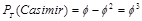(15)

exactly as found in earlier publications .

7. Discussion of a Paradigm Shift towards Empty Spacetime Vacuum

In the present paper we addressed many subjects that were wrapped together into a unity. This may be in some sense a paradigm shift taking Einstein’s insight of gravity being more or less the curvature of spacetime to its ultimate conclusion. Let us explain these main points in successive order:

A.  The most abundant matter in the universe is the non-materialist void around everything which we call empty space or real vacuum and which Einstein generalized to a geometrical manifold called spacetime.

B.  Spacetime is not just a stage where particles or particles and waves can perform their act nor is it a Pirandello play where audience, actors and Director interact together but far more than that. Cosmic spacetime on the quantum scale as well as on the Hubble scale is a unique theatre where everything is a by product of spacetime. In less prosaic terms, spacetime produces the quantum particle and the quantum wave and in turn the quantum particle and quantum waves produce spacetime and that in the most stringent mathematical meaning and not only as a metaphor.

C.  From our previous work and analysis we know that the photon has a fractal weight equal to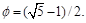This harmonizes fully with our Cantorian interval universe where the topological velocity of the light is exactly equal to. This topologicalis not only only the Hausdorff dimension of a cosmos made of a single random Cantor set, but it is also the topological velocity of light and the fractal weight of the photon.

D.  Witten’s M-theory is eleven dimensional. A fractal self-similar M-theory is consequently an eleven dimensional ‘cube’ inside another eleven dimensional cube and so on, indefinitely. This leads to a dimension equal to 11 plus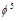whereis nothing but Hardy’s quantum entanglement which was proven to be accurate both theoretically as well as experimentally. In other words the probability of finding a ‘quantum’ point in 11 +is clearly the reciprocal value, namely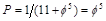. On the other hand the probability of not finding any is clearly equal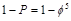. This shows that the average probability is simply the definite probability of one of the two outcomes of any measurement, namely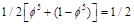. Remembering our analysis of ordinary energy density and dark energy density of the cosmos we see that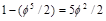is the topological dimensionless dark energy of the cosmos leading to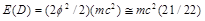and the ordinary energy density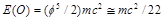.

The sum is of course E(Einstein) = mc2.

E.  From the fact that E(O) = mc2/2 and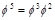wherewe see thatis in fact the topological mass and thus the Casimir latent topological energy is the mass of, if you want, the topological Higgs field given as ordinary measurable mass or ordinary measurable energy which on this deep level are one and the same thing. Extending the same idea we see that the topological mass of the dark energy is 5 and consequently the topological dark energy is (5)(). The average of both leads us again to Einstein’s formula.

F.   We surmised long ago that gravity is due to the passing of fractal time. Add this to the concept that there can be a Casimir force due to a gravity field and we end with a unified picture of all fundamental forces and the origin of all types of energies being spacetime vacuum and nothing else.

G.  Noting that cyrstalines increase the Casimir attraction we note that Fullerene nano particles can do this even better because the flat faces resemble mini Casimir channels and are currently our conjectured candidate for a nano Casimir reactor pending some actual experimental trials.

The above conclusions are presented once more in a condensed pictural and mathematical fashion in Fig. 1 and its lengthy legend.

8. Conclusion

Self similar quasi-crystal fractals manifest themselves to us on the largest as well as the smallest scales in nature. The cosmic fractal spacetime plasma and the quarks-gluon plasma are but an expression of this scale relativity theory [1-9]. These ideas are not entirely new and were considered before by many authors including Nottale, Ord, Finkelstein and Penrose [4,7,10,12]. What is new is our ability to calculate these vital physical effects connected to the big picture. Our model is really the simplest that there is because it starts with the basics of mathematics which is in turn the base of physics, namely set theory. Only three sets are needed in our present theory, the zero set, the empty set and the emptier still set. That way we have three concentric circles. The first is the particle with 0 andas bi-dimensions, the second is the surrounding circle which is the empty set with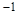andas their dimensions and then the infinitely large surrounding circle to average expectation bi-dimensions for infinitely many sets or multi-fractal given by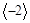and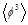expectation values. That means our Cantorian plasma picture leads to a spacetime picture of a particle wave set floating in a space made of the surface of the particle wave in monadic form. Putting this "flat" universe in five dimensions leads us to the correct ordinary and dark energy density of the cosmos as well as to understanding the zero point vacuum energy to the extent that we can start thinking seriously about harnessing it using nanotechnology .

References

1. R. Ent, T. Ulrich and R. Venugopalau: The glue that binds us. Scientific American, 312(5), May 2015, pp. 32-39.
2. M. Plumer, S. Raha and R. Weiner: How free is the quark-gluon plasma. Nukl. Phys. A, 418, 1984, pp. 549-557.
3. W.A. Zajc: The fluid nature of quark-gluon plasma. Nuclear Physics A, 805, 2008, p. 283-249.
4. M.S. El Naschie: A review of E-infinity theory and the mass spectrum of high energy physics. Chaos, Solitons & Fractals, 2004, 19(1), pp. 209-236.
5. M.S. El Naschie: Superstrings, knots and noncommutative geometry in E-infinity space. International Journal of Theoretical Physics, 37(12), 1998, pp. 2935-2951.
6. Mohamed S. El Naschie: Quantum Entanglement as a Consequence of a Cantorian Micro Spacetime Geometry. Journal of Quantum Information Science, 1(2), 2011, pp. 50-53.
7. M.S. El Naschie: The theory of Cantorian spacetime and high energy particle physics (An informal review). Chaos, Solitons & Fractals, 41(5), 2009, pp. 2635-2646.
8. Mohamed S. El Naschie: Kerr black hole geometry leading to dark matter and dark energy via E-infinity theory and the possibility of a nano spacetime singularity reactor. Natural Science, 7(4), 2015, pp. 210-225.
9. Ji-Huan He, M.S. El Naschie: On the monadic nature of quantum gravity as a highly structured golden ring, spaces and spectra. Fractal Spacetime and Noncommutative Geometry in Quantum and High Energy Physics. 2(2), 2012, pp. 94-98.
10. A. Connes: Noncommutative Geometry. Academic Press, San Diego, USA, 1994.
11. J. Polchinski: String Theory. Cambridge University Press. Cambridge 1998.
12. R. Penrose: The Road to Reality. A complete guide to the laws of the universe. Jonathan Cape, London. (2004).
13. M. Kaku: Introduction to Superstrings and M-theory. Springer, New York (1999).
14. Mohamed S. El Naschie: Topological-Geometrical and Physical Interpretation of the Dark Energy of the Cosmos as a ‘Halo’ Energy of the Schrodinger Quantum Wave. Journal of Modern Physics, 4(5), 2013, pp. 591-596.
15. D.R. Finkelstein: Quantum relativity. A synthesis of the ideas of Einstein and Heisenberg. Springer, Berlin, Germany, 1996.

 Contents 1. 2. 3. 4. 5. 6. 7. 8.
Article ToolsAbstractPDF(268K)